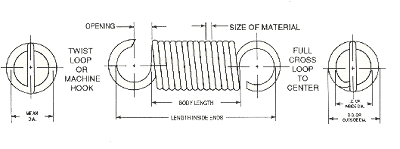# Design Considerations for Extension Springs

Extension Springs are springs which absorb and store energy by offering a resistance to a pulling force. Various type of ends are used to attach the Extension Spring to the source of the force.## Initial Tension

Most Extension Springs are wound with an initial tension. This is an internal force that holds the coils tightly wound together. The measure of the initial tension is the load required to overcome the internal force and just start coil separation.

The value for initial tension can be measured , but for our purposes it is sufficient to know that it exists and is supplied as one of the properties of each Extension Spring we specify.

## Spring rate = lbs. of load per inch of deflection

1. The spring rate is measured by extending the coil to a length (L1) such that coil separation occurs and measure the load (P1).
2. Extend the spring further to a second length (L2) and again measure the load (P2)
3. Calculate the load by dividing the load difference by the length difference in the following equation. R =(P2 - P1)/(L2 - L1).

## Extension Spring Ends

There are a variety of ends that can be put on an Extension Spring. By far the most common ends (shown in the diagram above) are the machine hook and full cross loop. These ends are made with standard tooling which minimizes the cost of producing the spring. Most Extension Spring failures occur at the ends. Failure rates will increase as the space occupied by the machine loop is shortened.